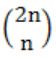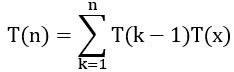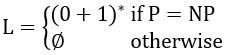## GATE 2003

 Question 1

Consider the following C function.

```float f(float x, int y)
{
float p, s; int i;
for (s=1, p=1, i=1; i < y; i ++)
{
p*= x/i;
s+=p;
}
return s;
}  ```

For large values of y, the return value of the function f best approximates

 A Xy B ex C ln(1+x) D Xx
Question 1 Explanation:
P = P * (x/i)
S = S+P
Iteration 1:
P=1; i=1; S=1
P=x
S = 1+x
Iteration 2:
P=x; S = 1+x; i=2
P = x * x/2 = x2/2
Iteration 3:
P = x2/2; S = 1+x+x2/2; i=3
P = (x2/2)(x/3) = x3/6
S = 1 + x + x2/2 + x3/6
Continue upto n
Then f( ) returns
:
S = 1 + x/1 + x2/2⋅1 + x3/3⋅2 + ...
= 1 + x/1! + x2/2! + x3/3! + ... + xn/n!
= ex
 Question 2

Assume the following C variable declaration

`int *A , B; `

Of the following expressions

`I. A     II. A     III. B     IV. B  `

which will not give compile-time errors if used as left hand sides of assignment statements in a C program?

 A I, II, and IV only B II, III, and IV only C II and IV only D IV only
Question 2 Explanation:
i) A can be consider as a pointer and this will not give any compile-time error.
ii) A This results an integer, no error will come.
iii) B is a base address of an array. This will not be changed it will result a compile time error.
iv) B This also results an integer. No error will come.
 Question 3

Let P(E) denote the probability of the event E. Given P(A) = 1, P(B) = 1/2, the values of P(A|B) and P(B|A) respectively are

 A 1/4, 1/2 B 1/2, 1/4 C 1/2, 1 D 1, 1/2
Question 3 Explanation:
P(A)=1, P(B)=1/2
P(A/B) = P(A∩B)/P(B)
= P(A)⋅P(B)/P(B) (consider P(A), P(B) are two independent events)
= 1
P(B/A) = P(B∩A)/P(A)
= P(B)⋅P(A)/P(A)
= 1/2
 Question 4

Let A be a sequence of 8 distinct integers sorted in ascending order. How many distinct pairs of sequences, B and C are there such that (i) each is sorted in ascending order, (ii) B has 5 and C has 3 elements, and (iii) the result of merging B and C gives A?

 A 2 B 30 C 56 D 256
Question 4 Explanation:
A can have sequence of 8 distinct integers which are sorted in ascending order.
→ If we are pick 3 elements from 8 sequence integers then remaining 5 elements are already in ascending order. After merging these elements then it gives A.
→ No. of possibilities of choosing 8 elements from total of 8 = 8C3
= 8!/3!5!
= 8 * 7
= 56
 Question 5

n couples are invited to a party with the condition that every husband should be accompanied by his wife. However, a wife need not be accompanied by her husband. The number of different gatherings possible at the party is

 ABCDQuestion 5 Explanation:
The possibilities to attend party is
i) Both husband and wife comes
ii) Only wife comes
iii) Both are not come
The no. of different gatherings possible at party is
= 3 * 3 * 3 * 3 * ... n times
= 3n
 Question 6

Let T(n) be the number of different binary search trees on n distinct elements. Then, where x is

 A n – k + 1 B n – k C n – k – 1 D n – k – 2
Question 6 Explanation:
A binary search tree consists of n distinct elements. Let consider on left subtree, it consists of (k-1) elements. Then right subtree consists of (n-k) elements. From this we c an write recursive function as T(k-1)*(n-k) i.e.,Question 7

Consider the set ∑* of all strings over the alphabet ∑ = {0, 1}. ∑* with the concatenation operator for strings

 A does not form a group B forms a non-commutative group C does not have a right identity element D forms a group if the empty string is removed from Σ*
Question 7 Explanation:
In the concatenation '∊' is the identity element. And given one is not a group because no element has inverse element.
→ To perform concatenation with the given set can result a Monoid and it follows the property of closure, associativity and consists of identity element.
 Question 8

Let G be an arbitrary graph with n nodes and k components. If a vertex is removed from G, the number of components in the resultant graph must necessarily lie between

 A k and n B k – 1 and k + 1 C k – 1 and n – 1 D k + 1 and n – k
Question 8 Explanation:
While a vertex is removed from a graph then that can be itself be forms a new component. The minimum number of components is k-1.
If a vertex is removed then it results that all the components are also be disconnected. So removal can create (n-1) components.
 Question 9

Assuming all numbers are in 2's complement representation, which of the following numbers is divisible by 11111011?

 A 11100111 B 11100100 C 11010111 D 11011011
Question 9 Explanation:
Given: Binary numbers = 11111011
MSB bit is '1' then all numbers are negative
1's complement = 00000100
2's complement = 00000100 + 00000001 = 00000101 = -5
(A) 11100111 - (-25)10
(B) 11100100 - (-28)10
(C) 11010111 - (-41)10
(D) 11011011 - (-37)10
Answer: Option A (-25 is divisible by -5)
 Question 10

For a pipelined CPU with a single ALU, consider the following situations

```I. The j + 1-st instruction uses the result of the j-th instruction as an operand
II. The execution of a conditional jump instruction
III. The j-th and j + 1-st instructions require the ALU at the same time ```

Which of the above can cause a hazard?

 A I and II only B II and III only C III only D All the three
Question 10 Explanation:
I is belongs to the Data hazard.
II is belongs to the Control hazard.
III is belongs to the Structural hazard.
→ Hazards are the problems with the instruction pipeline in CPU micro architectures.
 Question 11

Consider an array multiplier for multiplying two n bit numbers. If each gate in the circuit has a unit delay, the total delay of the multiplier is

 A Θ (1) B Θ (log n) C Θ (n) D Θ (n2)
Question 11 Explanation:
Each bit in Multiplier is ANDed with a bit in Multiplicand which produce n n-bit numbers. The multiplication takes n units of time. The n n-bit numbers are added by using (n-1) n-bit adders. The time taken by (n-1) n-bit adders is k*(n-1) units.
The total time is n+kn-k = Θ(n)
 Question 12

Ram and Shyam have been asked to show that a certain problem Π is NP-complete. Ram shows a polynomial time reduction from the 3-SAT problem to Π, and Shyam shows a polynomial time reduction from Π to 3-SAT. Which of the following can be inferred from these reductions?

 A Π is NP-hard but not NP-complete B Π is in NP, but is not NP-complete C Π is NP-complete D Π is neither NP-hard, nor in NP
Question 12 Explanation:
Note: As per Present syllabus, it is not required.
 Question 13

Nobody knows yet if P = NP. Consider the language L defined as follows:Which of the following statements is true?

 A L is recursive B L is recursively enumerable but not recursive C L is not recursively enumerable D Whether L is recursive or not will be known after we find out if P = NP
Question 13 Explanation:
Here, we have two possibilities, whether
P = NP (or) P != NP
→ If P=NP then L=(0+1)* which is regular, then it is recursive.
→ If P!=NP then L becomes ɸ which is also regular, then it is recursive.
So, finally L is recursive.
 Question 14

The regular expression 0*(10*)* denotes the same set as

 A (1*0)*1* B 0+(0+10)* C (0+1)*10(0+1)* D None of the above
Question 14 Explanation:
Both (A) and the given expression generates all strings over Σ.
Option (B) and (C) doesn't generate 11.
 Question 15

If the strings of a language L can be effectively enumerated in lexicographic (i.e., alphabetic) order, which of the following statements is true?

 A L is necessarily finite B L is regular but not necessarily finite C L is context free but not necessarily regular D L is recursive but not necessarily context free
Question 15 Explanation:
The given language L is to be recursively enumerable. The TM which accepts the language which is in lexicographic order. If the language is not in lexicographic order which is not accepted by TM.
The give 'L' is recursive but not necessarily context free.
 Question 16

Which of the following suffices to convert an arbitrary CFG to an LL(1) grammar?

 A Removing left recursion alone B Factoring the grammar alone C Removing left recursion and factoring the grammar D None of the above
Question 16 Explanation:
Left recursion removing (or) factoring the given grammar are not sufficient to convert an arbitrary CFG to an LL(1) grammar.
To convert an arbitrary CFG to an LL(1) grammar we need to remove the left recursion and as well as left factoring without that we cannot convert.
 Question 17

Assume that the SLR parser for a grammar G has n1 states and the LALR parser for G has n2 states. The relationship between n1 and n2 is:

 A n1 is necessarily less than n2 B n1 is necessarily equal to n2 C n1 is necessarily greater than n2 D None of the above
Question 17 Explanation:
No. of states in SLR and LALR are equal and no. of states in SLR and LALR are less than or equal to LR(1).
 Question 18

In a bottom-up evaluation of a syntax directed definition, inherited attributes can

 A always be evaluated B be evaluated only if the definition is L-attributed C be evaluated only if the definition has synthesized attributes D never be evaluated
Question 18 Explanation:
L-Attributed grammar can able to inherits either inherited attributes (or) synthesized attributes.
L-Attributed definitions are a class of syntax directed definitions whose attributes can be evaluated by a single traversal of the parse-tree.
 Question 19

Suppose the numbers 7, 5, 1, 8, 3, 6, 0, 9, 4, 2 are inserted in that order into an initially empty binary search tree. The binary search tree uses the usual ordering on natural numbers. What is the in-order traversal sequence of the resultant tree?

 A 7 5 1 0 3 2 4 6 8 9 B 0 2 4 3 1 6 5 9 8 7 C 0 1 2 3 4 5 6 7 8 9 D 9 8 6 4 2 3 0 1 5 7
Question 19 Explanation:Inorder: 0 1 2 3 4 5 6 7 8 9
 Question 20

Consider the following three claims

```I. (n + k)m = Θ(nm), where k and m are constants
II. 2n+1 = O(2n)
III. 22n+1 = O(2n) ```

Which of these claims are correct?

 A I and II B I and III C II and III D I, II, and III
Question 20 Explanation:
I) (n+k)m = Θ(nm)
Which is true by considering leading ordered term present in polynomial expression.
II) 2n+1 = Θ(nm) → True2n×2n can't be written as Θ(2n)
So, this is False.
 Question 21

Consider the following graphAmong the following sequences:

`(I) a b e g h f    (II) a b f e h g    (III) a b f h g e    (IV) a f g h b e `

Which are depth first traversals of the above graph?

 A I, II and IV only B I and IV only C II, III and IV only D I, III and IV only
Question 21 Explanation:
I) a → b → e → g → h → f (✔️)
II) a → b → f → e (✖️)
III) a → b → f → h → g → e (✔️)
IV) a → f → g → h → b → e (✔️)
 Question 22

The usual Θ(n2) implementation of Insertion Sort to sort an array uses linear search to identify the position where an element is to be inserted into the already sorted part of the array. If, instead, we use binary search to identify the position, the worst case running time will

 A remain Θ(n2) B become Θ(n (log n)2) C become Θ(n log n) D become Θ(n)
Question 22 Explanation:
While using Insertion sort to sort array by using linear search then time complexity = Θ(n2)
Instead, linear search use binary search then (log n) will be the worst case time complexity of binary search and performing n swaps to place an element in right position for the corresponding n elements
i.e., n×(logn+n)
Θ((n×logn)+n2)
Θ(n2)
Remains same.
There are 22 questions to complete.

Register Now